## Example Questions

← Previous 1

### Example Question #1 : How To Graph A Function

The Y axis is a _______________ of the function Y = 1/X

Equation

Zero solution

Vertical slope

Asymptote

Asymptote

Explanation:

A line is an asymptote in a graph if the graph of the function nears the line as X or Y gets larger in absolute value.

### Example Question #2 : How To Graph A Function

Which of the given functions is depicted below?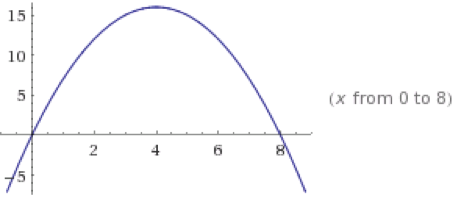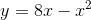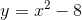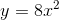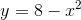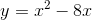Explanation:

The graph has x-intercepts at x = 0 and x = 8. This indicates that 0 and 8 are roots of the function.

The function must take the form y = x(x - 8) in order for these roots to be true.

The parabola opens downward, indicating a negative leading coefficient. Expand the equation to get our answer.

y = -x(x - 8)

y = -x2 + 8x

y = 8x - x2

Therefore, the answer must be y = 8x - x2

### Example Question #1 : How To Graph A Function

What is the domain of the following function: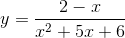x ≠ 5

x ≠ –1

x ≠ –2 and x ≠ –3

x = all real numbers

x ≠ 2

x ≠ –2 and x ≠ –3

Explanation:

The denominator cannot be zero, otherwise the function is indefinite. Therefore x cannot be –2 or –3.

### Example Question #4 : How To Graph A Function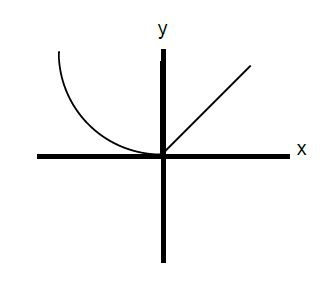The figure above shows the graph of y = f(x). Which of the following is the graph of y = |f(x)|?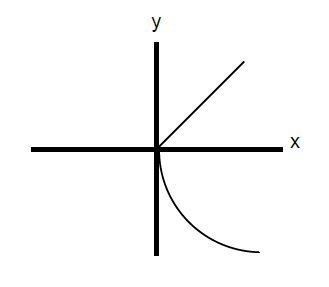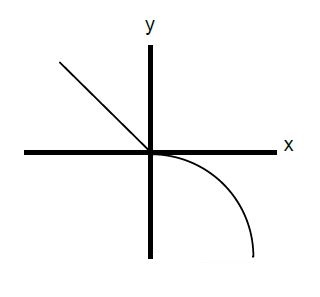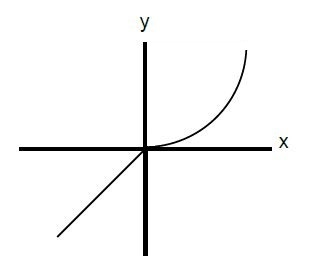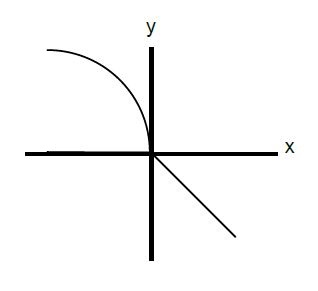Explanation:

One of the properties of taking an absolute value of a function is that the values are all made positive. The values themselves do not change; only their signs do. In this graph, none of the y-values are negative, so none of them would change. Thus the two graphs should be identical.

### Example Question #5 : How To Graph A Function

Below is the graph of the function:Which of the following could be the equation for?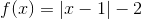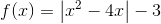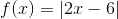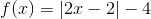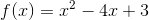Explanation:

First, because the graph consists of pieces that are straight lines, the function must include an absolute value, whose functions usually have a distinctive "V" shape. Thus, we can eliminate f(x) = x2 – 4x + 3 from our choices. Furthermore, functions with x2 terms are curved parabolas, and do not have straight line segments. This means that f(x) = |x2 – 4x| – 3 is not the correct choice.

Next, let's examine f(x) = |2x – 6|. Because this function consists of an abolute value by itself, its graph will not have any negative values. An absolute value by itself will only yield non-negative numbers. Therefore, because the graph dips below the x-axis (which means f(x) has negative values), f(x) = |2x – 6| cannot be the correct answer.

Next, we can analyze f(x) = |x – 1| – 2. Let's allow x to equal 1 and see what value we would obtain from f(1).

f(1) = | 1 – 1 | – 2 = 0 – 2 = –2

However, the graph above shows that f(1) = –4. As a result, f(x) = |x – 1| – 2 cannot be the correct equation for the function.

By process of elimination, the answer must be f(x) = |2x – 2| – 4. We can verify this by plugging in several values of x into this equation. For example f(1) = |2 – 2| – 4 = –4, which corresponds to the point (1, –4) on the graph above. Likewise, if we plug 3 or –1 into the equation f(x) = |2x – 2| – 4, we obtain zero, meaning that the graph should cross the x-axis at 3 and –1. According to the graph above, this is exactly what happens.

The answer is f(x) = |2x – 2| – 4.

### Example Question #6 : How To Graph A Function

Which of the following could be a value of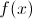for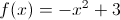?Explanation:

The graph is a down-opening parabola with a maximum of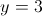. Therefore, there are no y values greater than this for this function.

### Example Question #1 : Graphing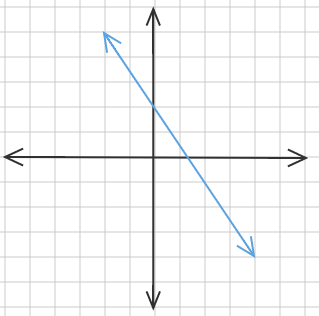What is the equation for the line pictured above?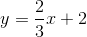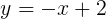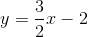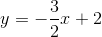Explanation:

A line has the equationwhereis theintercept andis the slope.

Theintercept can be found by noting the point where the line and the y-axis cross, in this case, at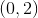so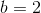.

The slope can be found by selecting two points, for example, the y-intercept and the next point over that crosses an even point, for example,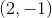.

Now applying the slope formula,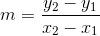which yields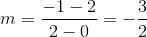.

Therefore the equation of the line becomes:### Example Question #8 : How To Graph A Function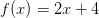Which of the following graphs represents the y-intercept of this function?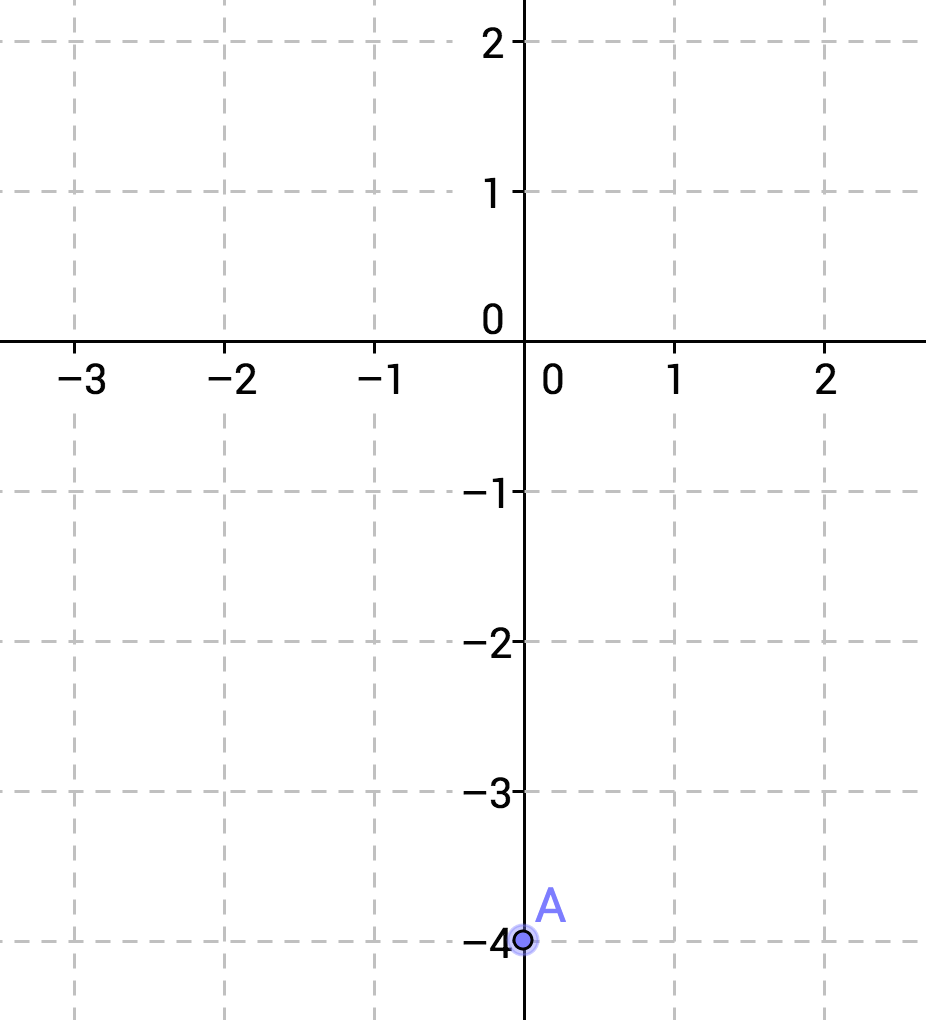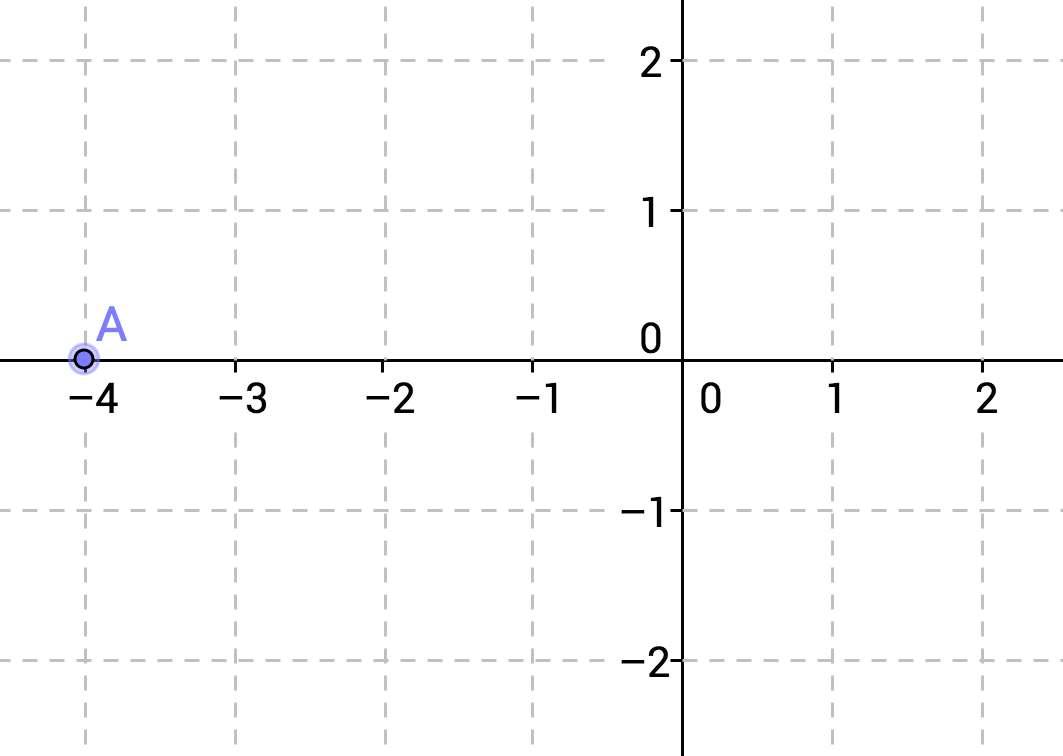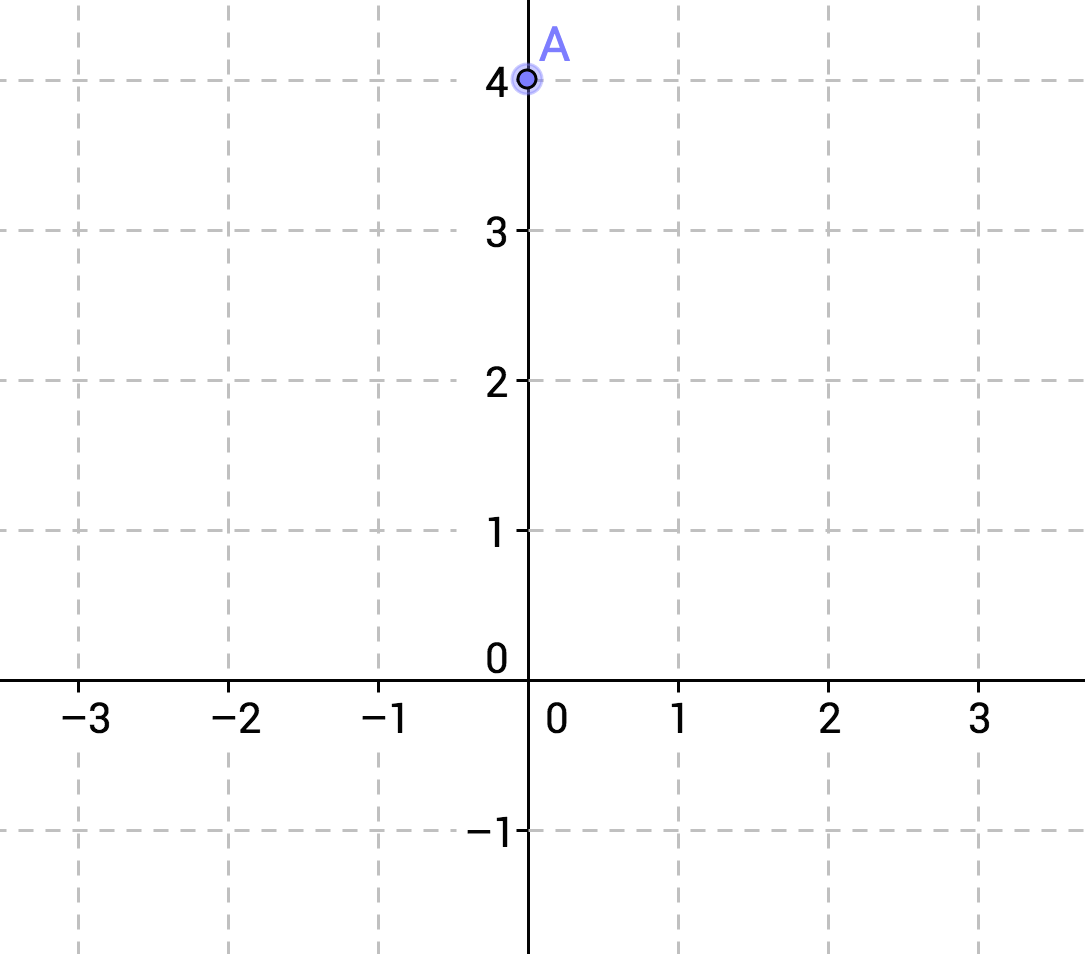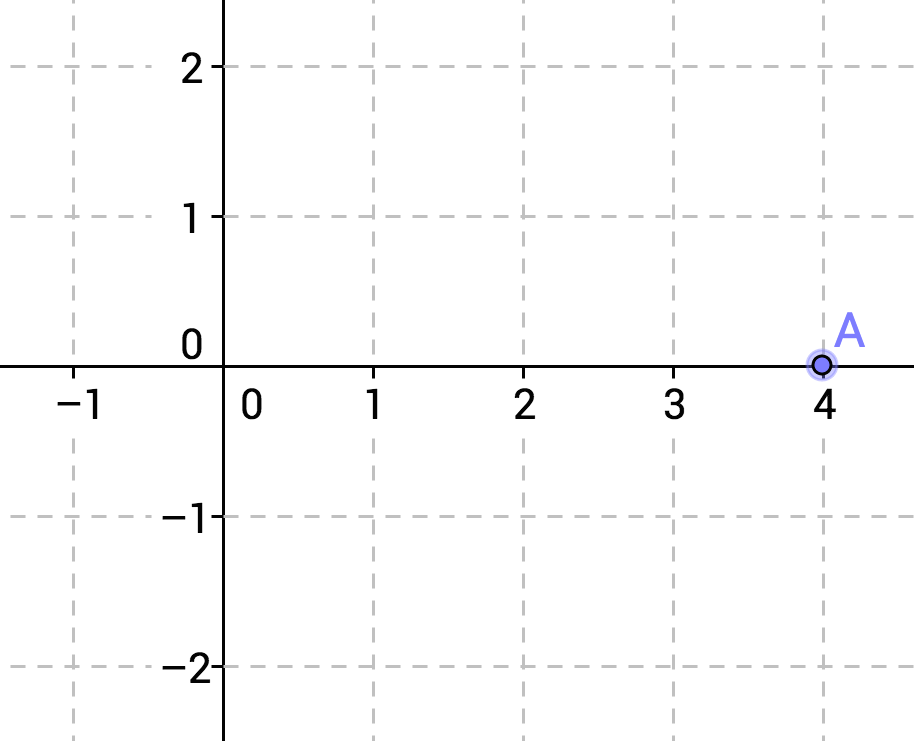Explanation:

Graphically, the y-intercept is the point at which the graph touches the y-axis.  Algebraically, it is the value of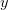when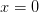.

Here, we are given the function.  In order to calculate the y-intercept, setequal to zero and solve for.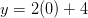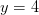So the y-intercept is at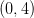.

### Example Question #9 : How To Graph A FunctionWhich of the following graphs represents the x-intercept of this function?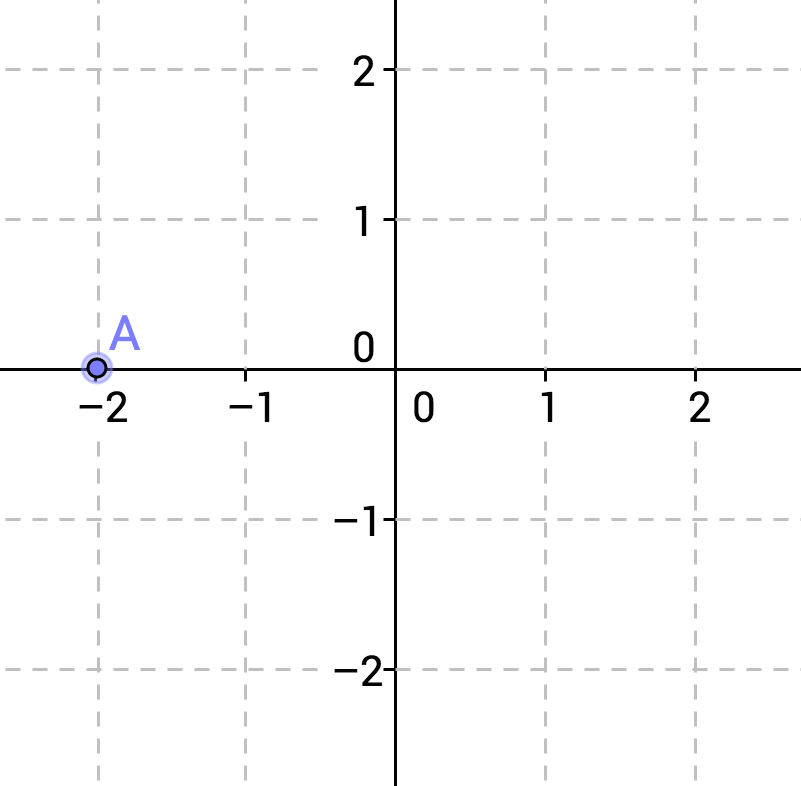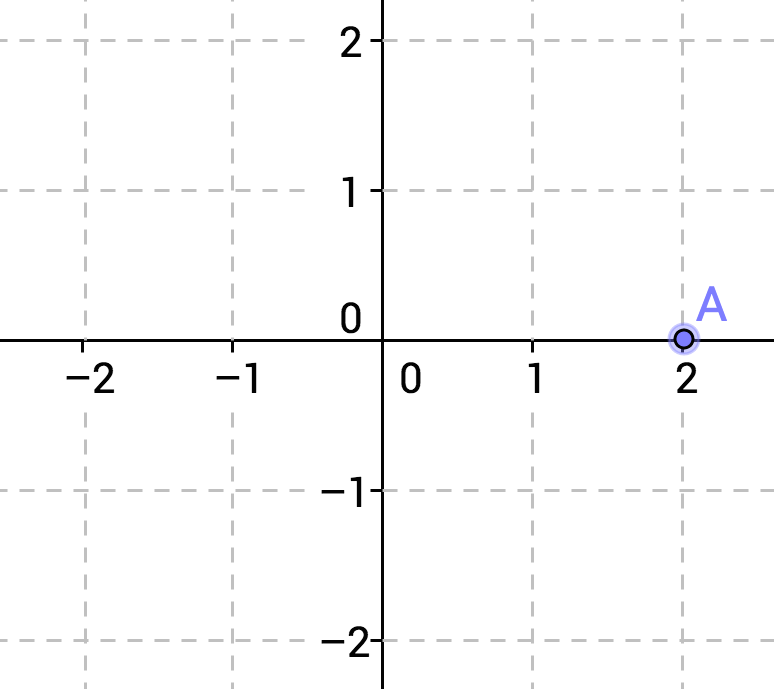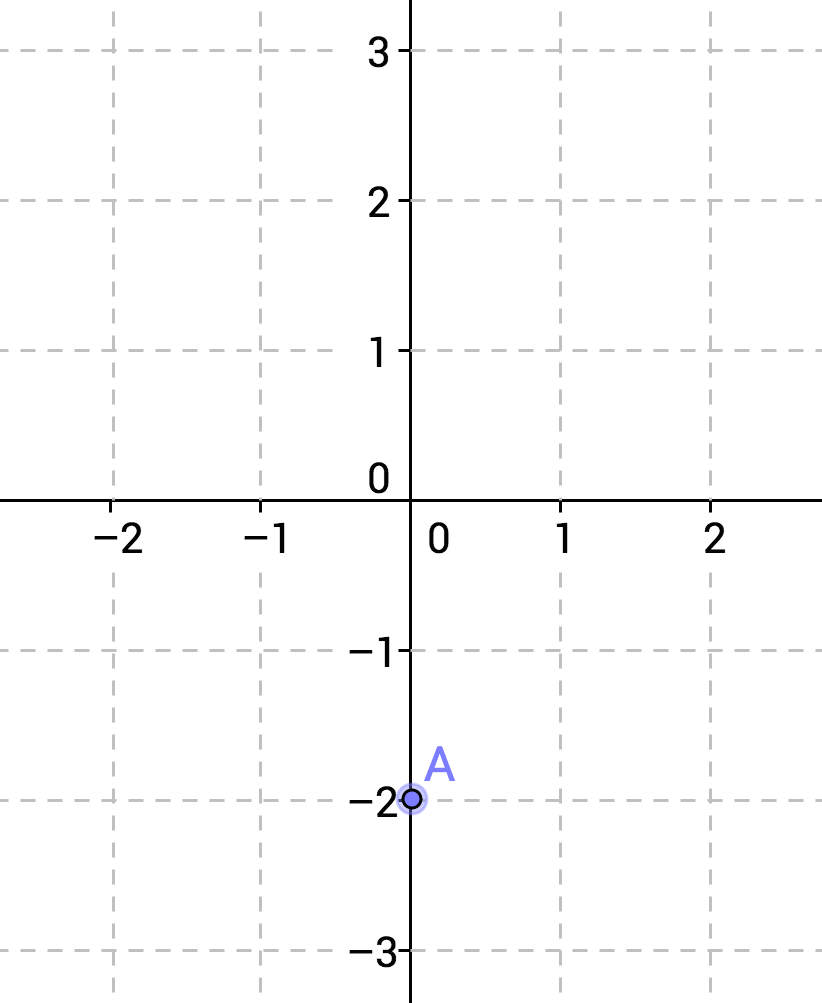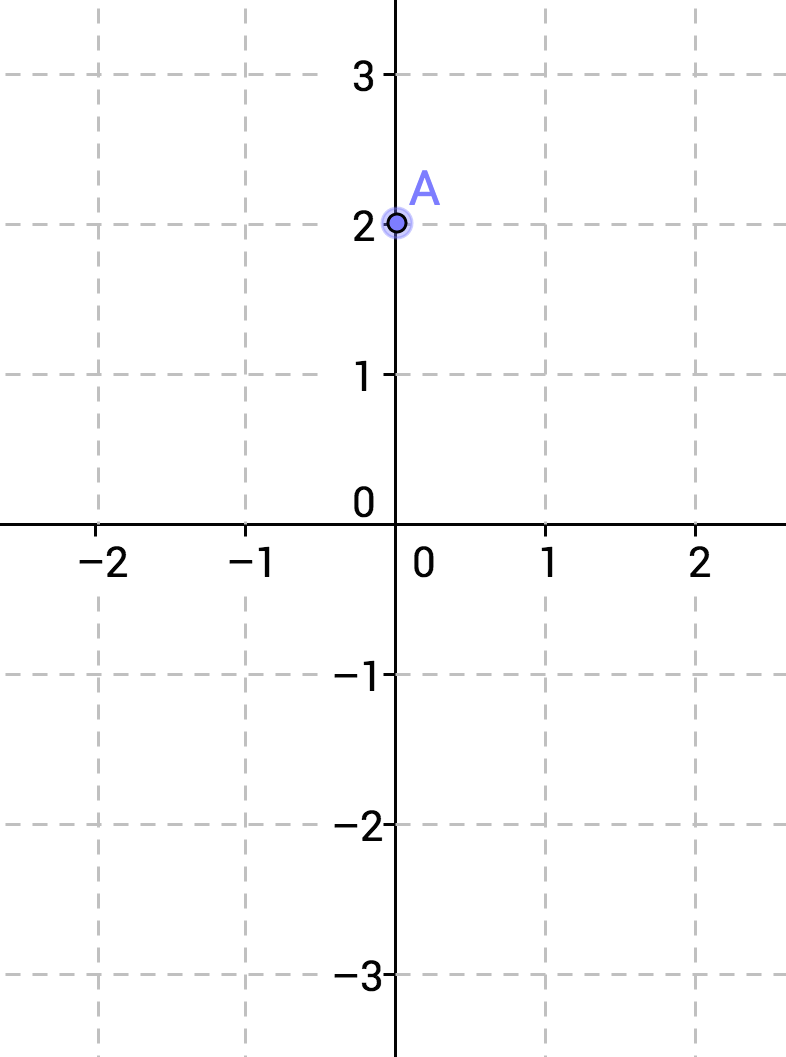Explanation:

Graphically, the x-intercept is the point at which the graph touches the x-axis.  Algebraically, it is the value offor which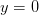.

Here, we are given the function.  In order to calculate the x-intercept, setequal to zero and solve for.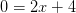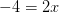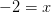So the x-intercept is at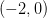.

### Example Question #10 : How To Graph A Function

Which of the following represents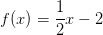?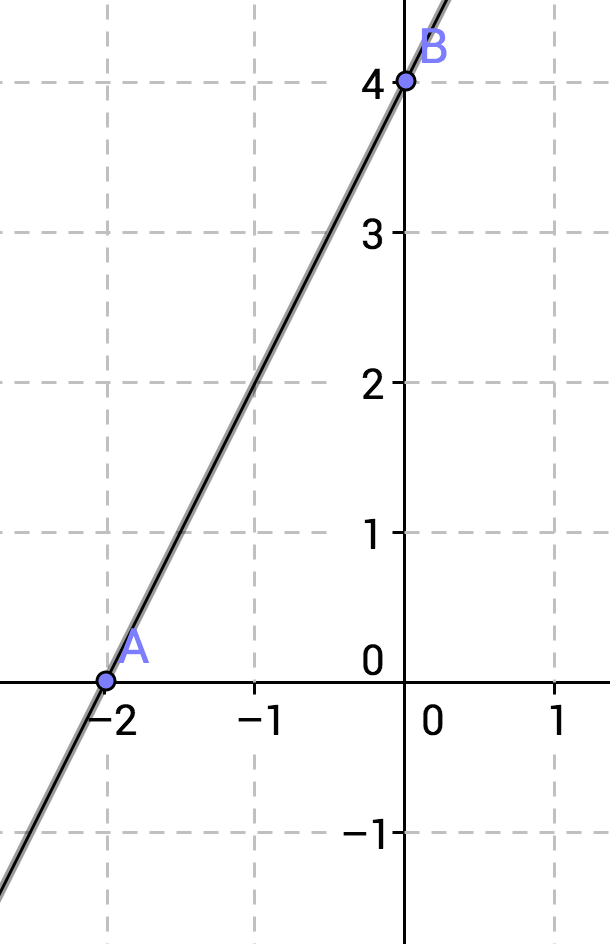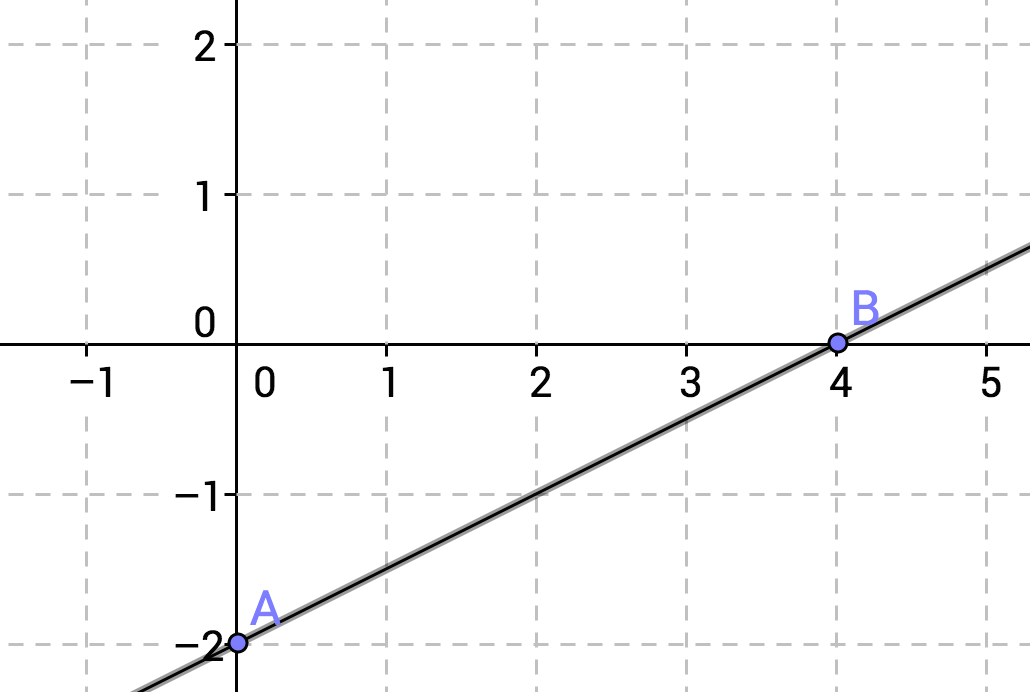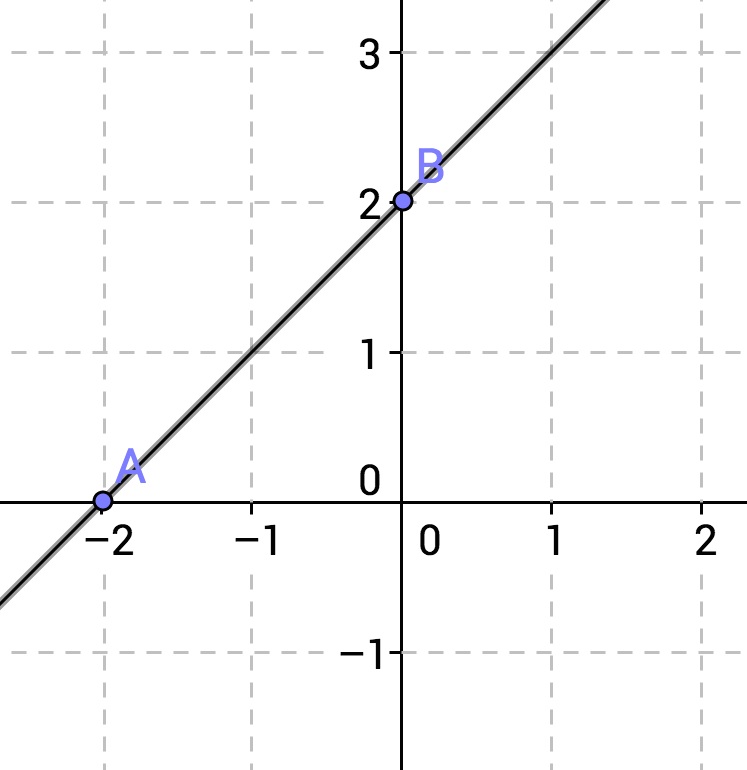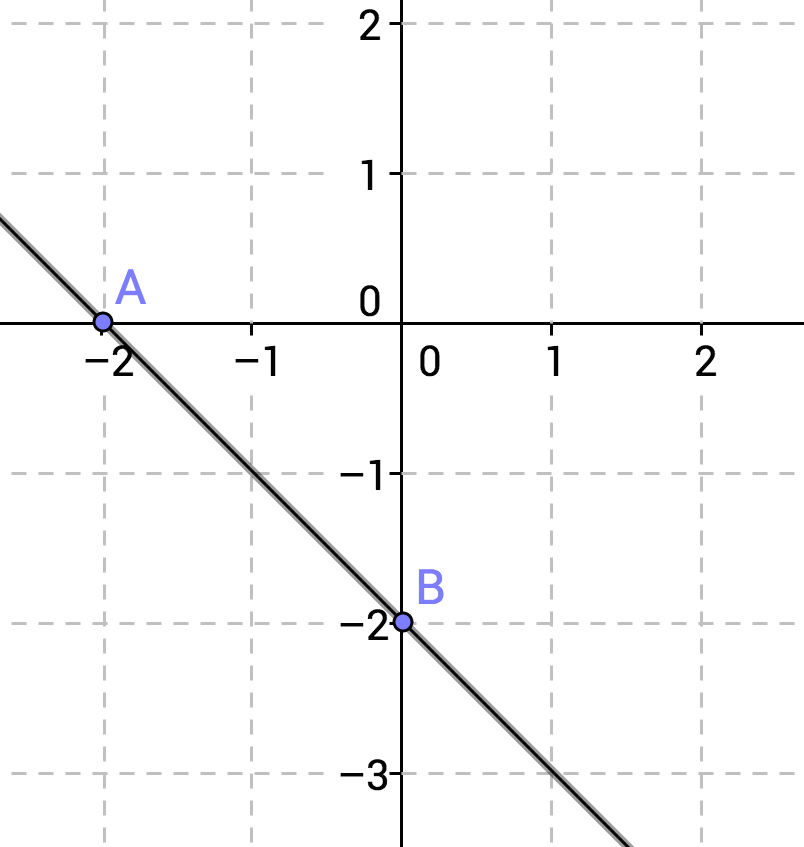Explanation:

A line is defined by any two points on the line.  It is frequently simplest to calculate two points by substituting zero for x and solving for y, and by substituting zero for y and solving for x.Let.  Then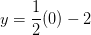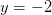So our first set of points (which is also the y-intercept) is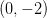Let.  Then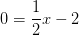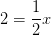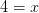So our second set of points (which is also the x-intercept) is.

← Previous 1